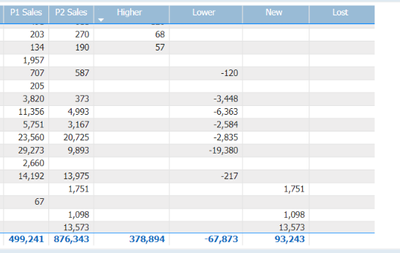cancel
Showing results for
Did you mean:Frequent Visitor

## Getting the Bucket Totals

Hello! I am comparing data from 2 periods by category and I managed to create measures and they look like this.Based on the values of P1 Sales and P2 Sales, the difference is put in the correct bucket (Higher, Lower, Lost, New)

However, at the Total Level, the total values of P1 and P2 sales are compared instead of the individual bucket totals, making all buckets empty except for Higher. How do I get the individual bucket totals?

``````Higher =
if([P2 Sales] > [P1 Sales] && not ISBLANK([P1 Sales])
, [P2 Sales] - [P1 Sales]
, BLANK()
)``````

``````Lower Sales =
if([P1 Sales] > [P2 Sales] && not ISBLANK([P2 Sales])
, [P2 Sales] - [P1 Sales]
, BLANK()
)<p> </p>``````

1 ACCEPTED SOLUTIONSuper User

@jmerencilla , Based on what I got, you have to force sume from visual row level

sumx(summarize(Table, Table[Visual column]),calculate(if([P2 Sales] > [P1 Sales] && not ISBLANK([P1 Sales])
, [P2 Sales] - [P1 Sales]
, BLANK()
)) )

3 REPLIES 3Frequent Visitor

@amitchandak , Thank you for the solution. It works perfectly as expected. But for some reason, the same DAX does not work in the Lost column. Now everything in Lost column is blank.It should just be the opposite of the New column

``````New =
SUMX(
SUMMARIZE(
'Sales'
, 'Sales'[Category]
)
, CALCULATE(
if([P2 Sales] > 0 && ISBLANK([P1 Sales])
, [P2 Sales]
, BLANK()
)
) ``````
``````Lost =
SUMX(
SUMMARIZE(
'Sales'
, 'Sales'[Category]
)
, CALCULATE(
if([P1 Sales] > 0 && ISBLANK([P2 Sales])
, -[P1 Sales]
, BLANK()
)
)
)``````

Appreciate it if you could spare a minute to look into this.Frequent Visitor

I tried to simply display the P1 Sales and I got this weird result:``````YTD Lost Sales =
SUMX(
SUMMARIZE(
'Sales'
, 'Sales'[Category]
)
, CALCULATE([P1 Sales])
)``````

Notice that those categories with P2 sales, do not show any values at all when it should. But with the opposite logic for New Sales, it's displaying the value correctly.

Appreciate any input you guys can provide me. Thanks!Super User

@jmerencilla , Based on what I got, you have to force sume from visual row level

sumx(summarize(Table, Table[Visual column]),calculate(if([P2 Sales] > [P1 Sales] && not ISBLANK([P1 Sales])
, [P2 Sales] - [P1 Sales]
, BLANK()
)) )